Home MonkeyNotes Printable Notes Digital Library Study Guides Message Boards Study Smart Parents Tips College Planning Test Prep Fun Zone Help / FAQ How to Cite New Title Request

 3.4 Sum of exterior angles of a Polygon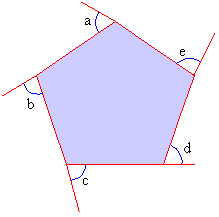Figure 3.4 Figure 3.4 shows a pentagon. Its external angles are named from a to e. The aim is to find the sum of these five angles. It is known that the sum of internal angles of a Pentagon = (5 - 2 ) ´ 1800 = 3 ´ 1800 = 540 \ each interior angle of the pentagon measures 5400 / 5 = 1080 The interior and exterior angles form linear pairs and hence are supplementary. \ Each exterior angle measures 1800 - 1080 = 720 \ Sum of five exterior angles = 5 ´ 72 = 3600 It can be proved that the sum of the exterior angles for any polygon is 360 0. Sum of interior angles of an n sided polygon = ( n - 2 ) 1800. \ Measure of each internal angle =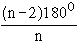\ Each exterior angle =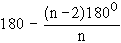\ Sum of n exterior angles =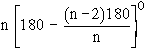=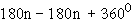=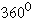Conclusion : The sum of interior angles of a polygon is dependent on the number of sides but the sum of the exterior angles is always 3600. Index 3. 1 Definition 3. 2 Terminology 3. 3 Sum Of Interior Angles Of A Polygon 3. 4 Sum Of Exterior Angles Of A Polygon 3. 5 Trapezoids 3. 6 Parallelogram 3. 7 Square, Rectangle And Rhombus Chapter 4
 Search: All Products Books Popular Music Classical Music Video DVD Toys & Games Electronics Software Tools & Hardware Outdoor Living Kitchen & Housewares Camera & Photo Cell Phones Keywords: